##### The Japanese Bonsai specialist
Direct order Contact Help / Services Newsletter# Table orientable

› Bonsai tools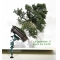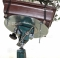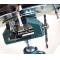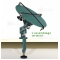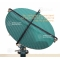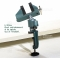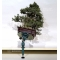ref. : 10023128,00

Available quantity : 3Order

###### Description

Rotating work tray for Bonsai that you can fix to a table thanks to its robust vice wrench ( opening of the jaws : 50 mm).

It enables to rotate very easily the bonsai on it in all directions ( very pleasant, especially during ligature hanging or specifics cares).

A diameter of 215 mm (covered by a skid-proof structure) on which, thanks to strong anchor points, you can easily attach small and medium sized bonsai.

This tray (that can be divided) is fixed to the vice wrench by a very robust ball point.

The whole of it is made with injected cast aluminium painted in the oven.

Sold without rubber tensioners and without the bonsai.

#bonsai 6.6 #table 3.5 #very 3.4 #orientable 3 #without 2.7 #wrench 2.6 #easily 2.6 #thanks 2.6 #robust 2.6 #tools 2.5

Formule
(( ROUND((CHAR_LENGTH(b.article_nom)-CHAR_LENGTH(REPLACE(b.article_nom, 'bonsai', '')))/LENGTH('bonsai')) + ROUND((CHAR_LENGTH(b.article_description)-CHAR_LENGTH(REPLACE(b.article_description, 'bonsai', '')))/LENGTH('bonsai')) ) * 4.6) + (( ROUND((CHAR_LENGTH(b.article_nom)-CHAR_LENGTH(REPLACE(b.article_nom, 'table', '')))/LENGTH('table')) + ROUND((CHAR_LENGTH(b.article_description)-CHAR_LENGTH(REPLACE(b.article_description, 'table', '')))/LENGTH('table')) ) * 3.5) + (( ROUND((CHAR_LENGTH(b.article_nom)-CHAR_LENGTH(REPLACE(b.article_nom, 'very', '')))/LENGTH('very')) + ROUND((CHAR_LENGTH(b.article_description)-CHAR_LENGTH(REPLACE(b.article_description, 'very', '')))/LENGTH('very')) ) * 3.4) + (( ROUND((CHAR_LENGTH(b.article_nom)-CHAR_LENGTH(REPLACE(b.article_nom, 'orientable', '')))/LENGTH('orientable')) + ROUND((CHAR_LENGTH(b.article_description)-CHAR_LENGTH(REPLACE(b.article_description, 'orientable', '')))/LENGTH('orientable')) ) * 3) + (( ROUND((CHAR_LENGTH(b.article_nom)-CHAR_LENGTH(REPLACE(b.article_nom, 'without', '')))/LENGTH('without')) + ROUND((CHAR_LENGTH(b.article_description)-CHAR_LENGTH(REPLACE(b.article_description, 'without', '')))/LENGTH('without')) ) * 2.7) + (( ROUND((CHAR_LENGTH(b.article_nom)-CHAR_LENGTH(REPLACE(b.article_nom, 'wrench', '')))/LENGTH('wrench')) + ROUND((CHAR_LENGTH(b.article_description)-CHAR_LENGTH(REPLACE(b.article_description, 'wrench', '')))/LENGTH('wrench')) ) * 2.6) + (( ROUND((CHAR_LENGTH(b.article_nom)-CHAR_LENGTH(REPLACE(b.article_nom, 'easily', '')))/LENGTH('easily')) + ROUND((CHAR_LENGTH(b.article_description)-CHAR_LENGTH(REPLACE(b.article_description, 'easily', '')))/LENGTH('easily')) ) * 2.6) + (( ROUND((CHAR_LENGTH(b.article_nom)-CHAR_LENGTH(REPLACE(b.article_nom, 'thanks', '')))/LENGTH('thanks')) + ROUND((CHAR_LENGTH(b.article_description)-CHAR_LENGTH(REPLACE(b.article_description, 'thanks', '')))/LENGTH('thanks')) ) * 2.6) + (( ROUND((CHAR_LENGTH(b.article_nom)-CHAR_LENGTH(REPLACE(b.article_nom, 'robust', '')))/LENGTH('robust')) + ROUND((CHAR_LENGTH(b.article_description)-CHAR_LENGTH(REPLACE(b.article_description, 'robust', '')))/LENGTH('robust')) ) * 2.6) + (( ROUND((CHAR_LENGTH(b.article_nom)-CHAR_LENGTH(REPLACE(b.article_nom, 'that', '')))/LENGTH('that')) + ROUND((CHAR_LENGTH(b.article_description)-CHAR_LENGTH(REPLACE(b.article_description, 'that', '')))/LENGTH('that')) ) * 2.4)

## Secure payment## Delivery

Our logistic partners :04 74 55 23 48
Pépinière MAILLOT-BONSAÏ
Le Bois Frazy
01990 RELEVANT - FRANCE
on appointment